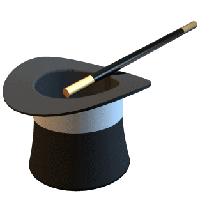## Exercises - Arguments and Expressions

Some of the exercises below provide sample runs to better clarify what the program in question is supposed to do. In these sample runs, text given in green has been typed by the user, while white text has been output by the program. Additionally, the "$" symbol indicates the command prompt, while the "↵" symbol indicates the user has pressed the return key. 1.  public class HelloWorld { public static void main(String[] args) { System.out.println("Hello World!"); } }  2.  public class TripleReverse { public static void main(String[] args) { System.out.println(args); System.out.println(args); System.out.println(args); } }  3. Write a class named Parrot that takes a single string command-line argument and "parrots" it back by printing it to the command line, followed by a "Squawk!" as shown in the two sample runs below. (Note, if the string you wish to pass as an argument to this program contains spaces, you must surround the string with double quotes.) $ java Parrot Arrrggh↵
Arrrggh, Squawk!

$java Parrot "Polly Wanna Cracker"↵ Polly Wanna Cracker, Squawk!  4. Write a class named SumFinder which takes two integer arguments and returns their sum. Below is a sample run: $ java SumFinder 13 7↵
20



public class SumFinder {

public static void main(String[] args) {

int a = Integer.parseInt(args);
int b = Integer.parseInt(args);
int sum = a + b;

System.out.println(sum);
}
}


5. Write a class named ProductFinder which takes three integer arguments and returns their product. Below is a sample run:

$java ProductFinder 2 3 5↵ 30  6.  public class FeetToOtherUnits { public static void main(String[] args) { double ft = Double.parseDouble(args); double in = 12 * ft; double cm = in / 2.54; System.out.println(ft + " feet, "); System.out.println("or equivalently " + in + " inches, "); System.out.println("or " + cm + " centimeters"); } }  7.  public class EggCartons { public static void main(String[] args) { int numEggs = Integer.parseInt(args); int cartonsNeeded = (numEggs - 1) / 12 + 1; System.out.println(cartonsNeeded + " carton(s) are needed"); } }  8. Recall, for a point with cartesian coordinates$(x,y)$, the corresponding point written in polar coordinates is given by$(r,\theta)$, where$r$is the distance from$(0,0)$to$(x,y)$and$\theta$is the measure of the angle formed between the positive$x$-axis and the ray from$(0,0)$to$(x,y)$. Further,$\theta$is positive when the angle is swept from the positive$x$-axis to the aforementioned ray in a counter-clockwise way, and negative otherwise. Write a class named CartesianToPolar that takes two (potentially decimal) values as command line arguments$x$and$y$, converts them to polar coordinates and displays the results in a manner consistent with the following sample runs. $ java CartesianToPolar 10 10↵
r = 14.14, θ = 45.00°

$java CartesianToPolar 1 5↵ r = 5.10, θ = 78.69°  $ java CartesianToPolar -3 -4↵
r = 5.00, θ = -126.87°


In particular, note the presence of special characters for theta and the degree symbol, and that values for r and theta are printed with two decimal places. (With regard to the printing numerical values to two decimal places, make sure to read about the printf method)

9. Write a class named MagicTrick that takes three distinct single digits as arguments, and then performs the following tasks:1. Form the unique three-digit number from the digits provided, where the digits of the number formed strictly decrease from left to right.

2. Find the number whose digits are the reverse of the number formed in the previous step.

3. Given the two numbers formed above, subtract the smaller one from the larger one.

4. Find the number whose digits are the reverse of the difference just found.

5. Add the last two numbers together (i.e., the difference and the "reversed" difference)

The numbers found above should be printed in a manner similar to that shown in the sample run below.

Run this program several times, starting with different sets of three distinct single digit arguments -- do you notice anything seemingly magical? Why does this happen?

Below is a sample run:

\$ java MagicTrick 5 4 7↵
Number: 754
754 - 457 = 297
297 + 792 = 1089


To accomplish some of the tasks above, you may find the functions Math.min() and Math.max() very useful. For more information, see Commonly Used Methods of the java.lang.Math class.